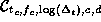Next: The pyramidal (multi-resolution) CCT Up: Multiple Mother Chirplets: The Previous: Pyramidal (multiresolution) true-rectangular TF

### Parallelogram-shaped tilings of the TF plane

The method of multiple windows may be extended further to the chirplet framework.

This further extension makes use of the same families of multiple windows that are used in the Thomson method, and that we first extended to the true rectangular tiling of the TF plane, but instead they will now be used within the context of the operators of Table 1. In the same way that the Thomson method consists of computing power spectra with a plurality of windows, and averaging the power spectra together, we compute the power CCTs with a plurality of windows, and average the results together. To compute an appropriately-smoothed version the chirplet transform, we compute a CCT (11) using each one of the multiple windows as the mother chirplet. We then average the squared-magnitudes of the resulting CCTs together, weighting by the eigenvalues, just as with the Thomson method. This gives us the CCT at a particular value of NW.

Alternatively, we may consider a given point in the five-dimensional CCT parameter space, say,, is given by applying the operatorto the set of multiple windows and then computing the sum of absolute squared energy:

S(t_c,f_c,(_t),c,d) = _i | C_t_c,f_c,(_t),c,d g_i(t) \: | s(t) |^2

We now refer to the multiple windows as ``multiple mother chirplets'' as they have collectively taken the role of the single mother chirplet. They act collectively to produce an idealized parallelogram-shaped smoothing of the TF (Wigner) distribution (Fig. 5), where the area of the parallelogram is NW.

For example, if we apply a frequency shear, with parameter c=0.85, to each of the mother chirplets, the new set of functions will collectively occupy the parallelogram-shaped region of the TF plane indicated in the Fig 5, lower right. This energy concentration represents a single point located at coordinates (0, 0, 0, 0.85, 0) in the averaged squared-magnitude CCT.

Figure: FIGURE GOES SOMEWHERE IN THIS GENERAL VICINITYNext: The pyramidal (multi-resolution) CCT Up: Multiple Mother Chirplets: The Previous: Pyramidal (multiresolution) true-rectangular TF

Steve Mann
Thu Jan 8 19:50:27 EST 1998